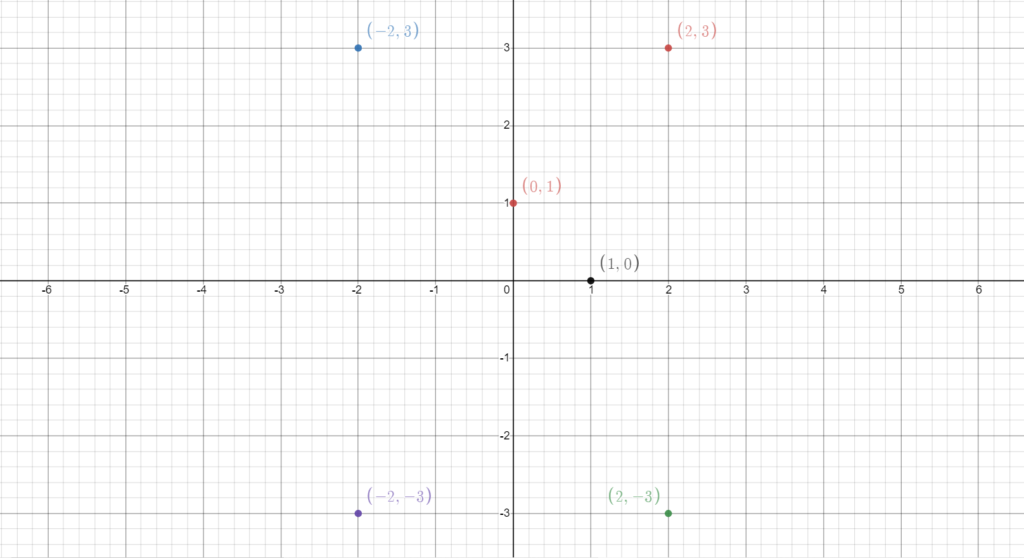# Complex Numbers

There are two primary approaches to introducing complex numbers to a newcomer:

1. Taking roots of negative numbers
2. Giving an idea of 2-dimensional numbers

I’ll follow the second approach since we don’t need much of this to have a basic understanding of complex numbers.

You must have heard of the real number line. Pause for a moment and ponder upon everything you know about this line. What is this line all about? What does this represent?

Basically, we are taking an infinitely long line and assigning a real number to each point on the line. But, we can do that with an infinite plane as well, isn’t it? We can take a plane and give each of its points a number using some suitable scheme.

That’s exactly what we do. We take two perpendicular axes (namely the X-axis and the Y-axis) to define the plane properly. Now, we will try to assign each point a duplet of numbers (in mathematical terminology, it’s called an ordered pair). We follow the following scheme:

• Take any point on the plane.
• Calculate its perpendicular distance from Y-axis and call it a (if the point is on the Y-axis, then a = 0).
• Follow the same procedure for the X-axis and get b.
• Assign the ordered pair (a, b) to this point.
• From now on, this point is named (a, b).

Note that the points on the X-axis are of the form (x, 0) and the points on the Y-axis are of the form (0, y).

###### Fun Exercise:

Try to give a geometric proof of:

1. Two points on a plane will never be assigned the same name.
2. A point on a plane cannot have more than one name.

Hint: Think about the properties of lines parallel to an axis

Well, that’s what I meant by two-dimensional numbers (since these numbers sit on a two-dimensional plane).

I know what you’re thinking. Yes, it’s true that we can define three-dimensional, four-dimensional or any dimensional numbers. But we don’t need them at least in the present discussion.

Note that the real numbers are also a part of the complex numbers. The X-axis is simply the real number line, i.e., the real number r has been renamed as (r, 0) according to the new scheme. Now, once we have defined them, we need to start working with them as well. So, we have defined addition and multiplication on them as well. The definitions are as follows-

(a1, b1) ± (a2, b2) = (a1 ± a2, b1 ± b2

(a1, b1).(a2, b2) = (a1a2 − b1b2, a2b1 + b2a1

Of course, there are motivations that lead us to these definitions, but I won’t talk about it now. Just remember what complex numbers are, and remember that almost all operations that we perform on real numbers are valid for complex numbers.

That’s all we’ll need to learn in this journey.Figure 1: Here are a few examples of complex numbers. Note that the point (1,0) is of the form (x,0) and hence sits on the X-axis, and the point (0,1) is of the form (0,y) and hence sits on the Y-axis.

Sum of Naturals